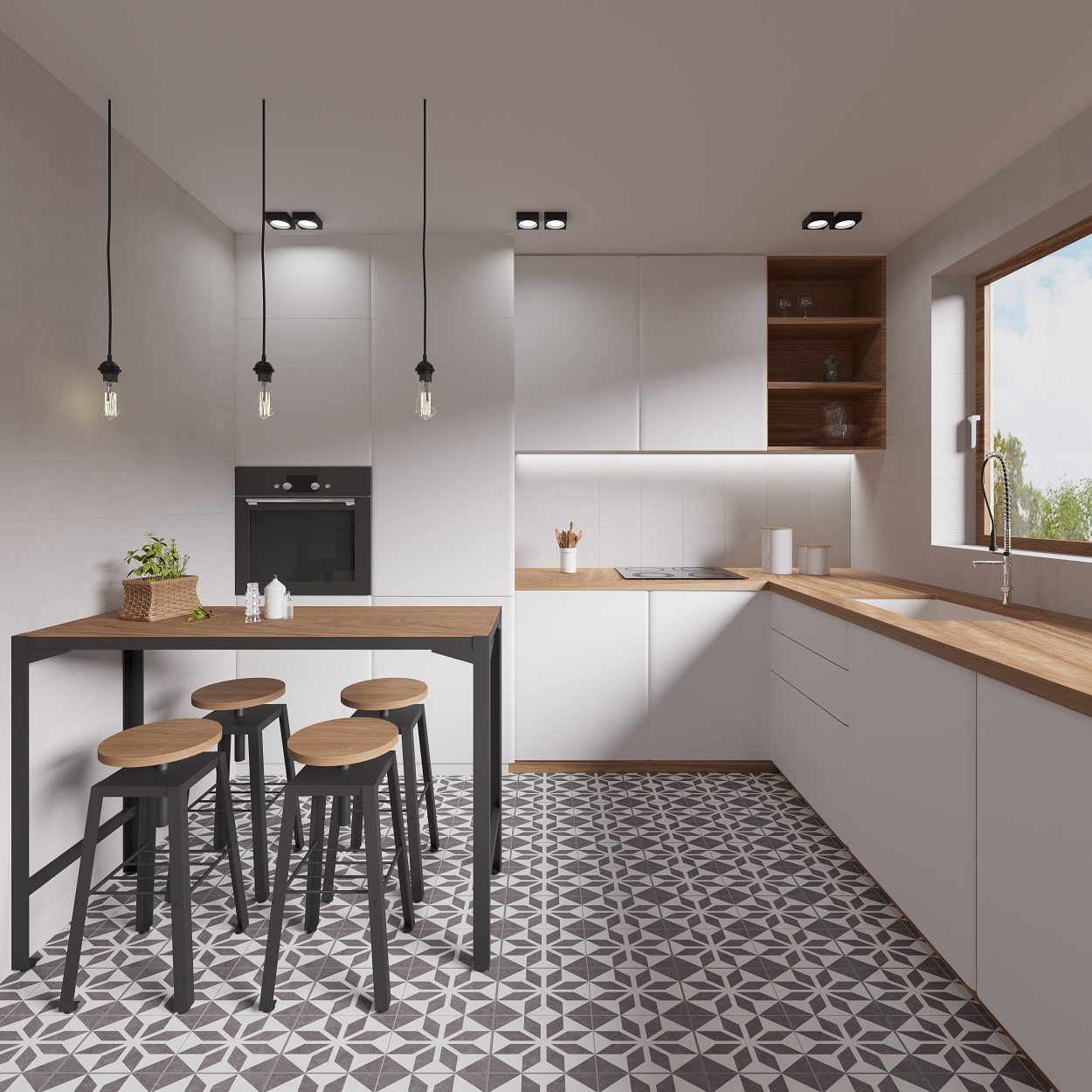top of pageSearch

# How to calculate how many tiles are needed for a project

One of the most important steps in any tiling project is calculating how many tiles are needed, and in our latest blog we take you through the key steps to follow to ensure you have the exact number of tiles needed.

## Step 1: Measure wall or floor areas

The first step is to measure your wall or floor areas, so you will need to have a tape measure, pencil, paper, and calculator to hand. You will need to measure both the length and width of each wall or floor that needs to be tiled, but remember to take into account obstructions such as windows and doors.

Square and rectangular areas are naturally easier to measure, but if you need to measure a more complicated space, simply divide the area into smaller rectangles and measure the width and length of each.

## Step 2: Multiply the measurements to find the total area

Once you have made a note of the length and width of each area, multiply them together to get the total area to be tiled. For example, if the floor measures 200cm wide and 300cm long, the total floor area would be 200cm x 300cm = 60,000cm² / 6m². If you are calculating the total area of a more complicated shape, you will need to complete this calculation for each square or rectangular box and then add the two together to find the total.

## Step 3: Calculate the overage required

The calculations above will determine the exact area size for tiling, but the measurements will not allow for potential breakages and offcuts. In most situations, an overage of 10% is usually enough to cover potential accidents, and it will also mean there are a few tiles spare to cover possible future breakages.

To find the overage, multiply the total area calculated in Step 2 by 10%. For example, in this situation the calculation would be 6m² x 1.1 = 6.6m².

## Step 4: Calculate how many tiles are needed

Firstly, you will need to check the measurements of your chosen tiles and how many come in a box. The number of tiles in a box can vary dramatically, with smaller subway style tiles having far more tiles per box than large format designs. Next, calculate the area covered per tile, so using the same calculation as above, if a tile measures 10cm x 20cm, the total tile area will be 200cm² / 0.02m².

Then, divide the total area of the room including overage by the total area covered by tile. In this example, the calculation would be 6.6m²/0.02m² = 330 tiles. Now, check how many tiles are included in a box and divide the total number of tiles required by the amount in each box. For example, if there are 20 tiles in a box, the calculation would be 330/20 = 16.5 boxes, which would need to be rounded up to 17 boxes.

In practice, many of the tile boxes will include details such as the area covered by each box or how many tiles will be needed to cover 1m².

## Navarro Tiles - Wholesale suppliers of high-quality tiles throughout the UK & Ireland

It is always worth remembering that walls are rarely perfectly straight and wastage can vary depending on patterns, tile size and layout. If in any doubt, always round your calculations up, as it can be difficult to find matching tiles from the same batch at a later date.

We hope the above steps prove to be useful when you calculate how many tiles are needed for your project. If you would like to find out more about our wide range of high-quality tiles, contact our team today.